# 7 The coupled theory: Lagrangian and gauge structure

In this section we start from the concrete form of (151) and identify the entire gauge structure of the Lagrangian model that describes all consistent interactions in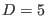between the BF theory and the massless tensor field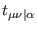. To this end we recall the discussion from the end of section 2 related to the relationship between the gauge structure of a given Lagrangian field theory and various terms of definite antighost number present in the solution of the master equation. Of course, we assume that the functions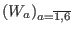,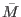together with the constants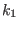and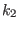satisfy equations (130)-(135) and (141)-(142). The analysis of solutions that are interesting from the point of view of cross-couplings (at least one of the constantsandis nonvanishing) is done in Section 8.

The piece of antighost number zero from (151) provides nothing but the Lagrangian action of the interacting theory(179)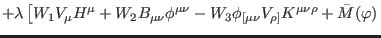(180)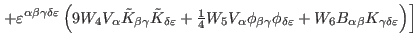(181)(182)(183)

where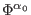is the field spectrum (2). The terms of antighost number one from the deformed solution of the master equation, generically written as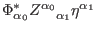, allow the identification of the gauge transformations of action (152) via replacing the ghostswith the gauge parameters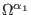(184)

In our case, taking into account formula (151) and maintaining the notation (8) for the gauge parameters, we find the concrete form of the deformed gauge transformations as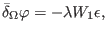(185)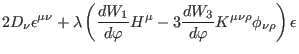(186)(187)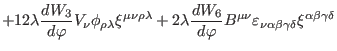(188)(189)(190)(191)(192)(193)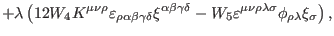(194)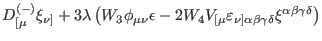(195)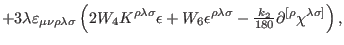(196)(197)(198)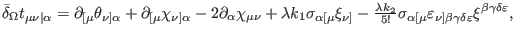(199)

where, in addition, we used the notations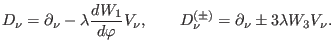(200)

We observe that the cross-interaction terms,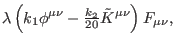are only of order one in the deformation parameter and couple the tensor fieldto the two-formand to the three-form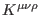from the BF sector. Also, it is interesting to see that the interaction components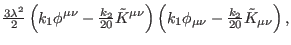which describe self-interactions in the BF sector, are strictly due to the presence of the tensor(in its absence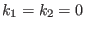, so they would vanish). The gauge transformations of the BF fieldsandare deformed in such a way to include gauge parameters from the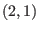sector. Related to the other BF fields,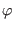,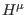,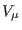, and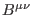, their gauge transformations are also modified with respect to the free theory, but only with terms specific to the BF sector. A remarkable feature is that the gauge transformations of the tensorare modified by shift terms in some of the gauge parameters from the BF sector.

From the components of higher antighost number present in (151 ) we read the entire gauge structure of the interacting theory: the commutators among the deformed gauge transformations (154)-(160), and hence the properties of the deformed gauge algebra, their associated higher-order structure functions, and also the new reducibility functions and relations together with their properties. (The reducibility order itself of the interacting theory is not modified by the deformation procedure and remains equal to that of the free model, namely, three.) We do not give here the concrete form of all these deformed structure functions, which is analyzed in detail in Appendix F, but only briefly discuss their main properties by contrast to the gauge features of the free theory (see section 2).

The nonvanishing commutators among the deformed gauge transformations result from the terms quadratic in the ghosts with pure ghost number one present in (151). Since their form can be generically written as, it follows that the commutators among the deformed gauge transformations only close on-shell (on the stationary surface of the deformed field equations)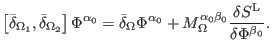(201)

Here,stand for the Euler-Lagrange (EL) derivatives of the interacting action (152),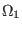andrepresent two independent sets of gauge parameters of the type (8), and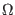is a quadratic combination ofand. The exact form of the corresponding commutators is included in the Appendix F (see formulas (287)-(293)). In conclusion, the gauge algebra corresponding to the interacting theory is open (the commutators among the deformed gauge transformations only close on-shell), by contrast to the free theory, where the gauge algebra is Abelian.

The first-order reducibility functions and relations follow from the terms linear in the ghosts for ghosts appearing in (151). Because they can be generically set in the form (, it follows that if we transform the gauge parametersin terms of the first-order reducibility parameters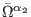as in(202)

then the transformed gauge transformations (153) of all fields vanish on-shell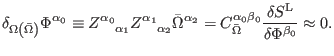(203)

Along the same line, the second-order reducibility functions and relations are given by the terms linear in the ghosts for ghosts for ghosts appearing in (151), which can be generically written as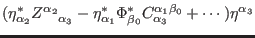. Consequently, if we transform the first-order reducibility parametersin terms of the second-order reducibility parameters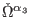as in(204)

then the transformed gauge parameters (163) vanish on-shell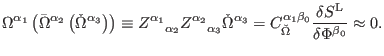(205)

Finally, the third-order reducibility functions and relations are withdrawn from the terms linear in the ghosts for ghosts for ghosts for ghosts from ( 151), which have the generic form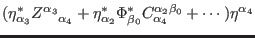, such that if we transform the second-order reducibility parametersin terms of the third-order reducibility parametersas in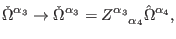(206)

then the transformed first-order reducibility parameters (165) again vanish on-shell(207)

In the above the notations,,, andare the same from the free case, namely (8), (16), (20), and (23), while the BRST generators are structured according to formulas (25)-(31). It is now clear that the reducibility relations associated with the interacting model ((164 ), (166), and (168)) only hold on-shell, by contrast to those corresponding to the free theory ((10), (12), and respectively (14)), which hold off-shell. Their concrete form is detailed in Appendix F.

Ashkbiz Danehkar
2018-03-26